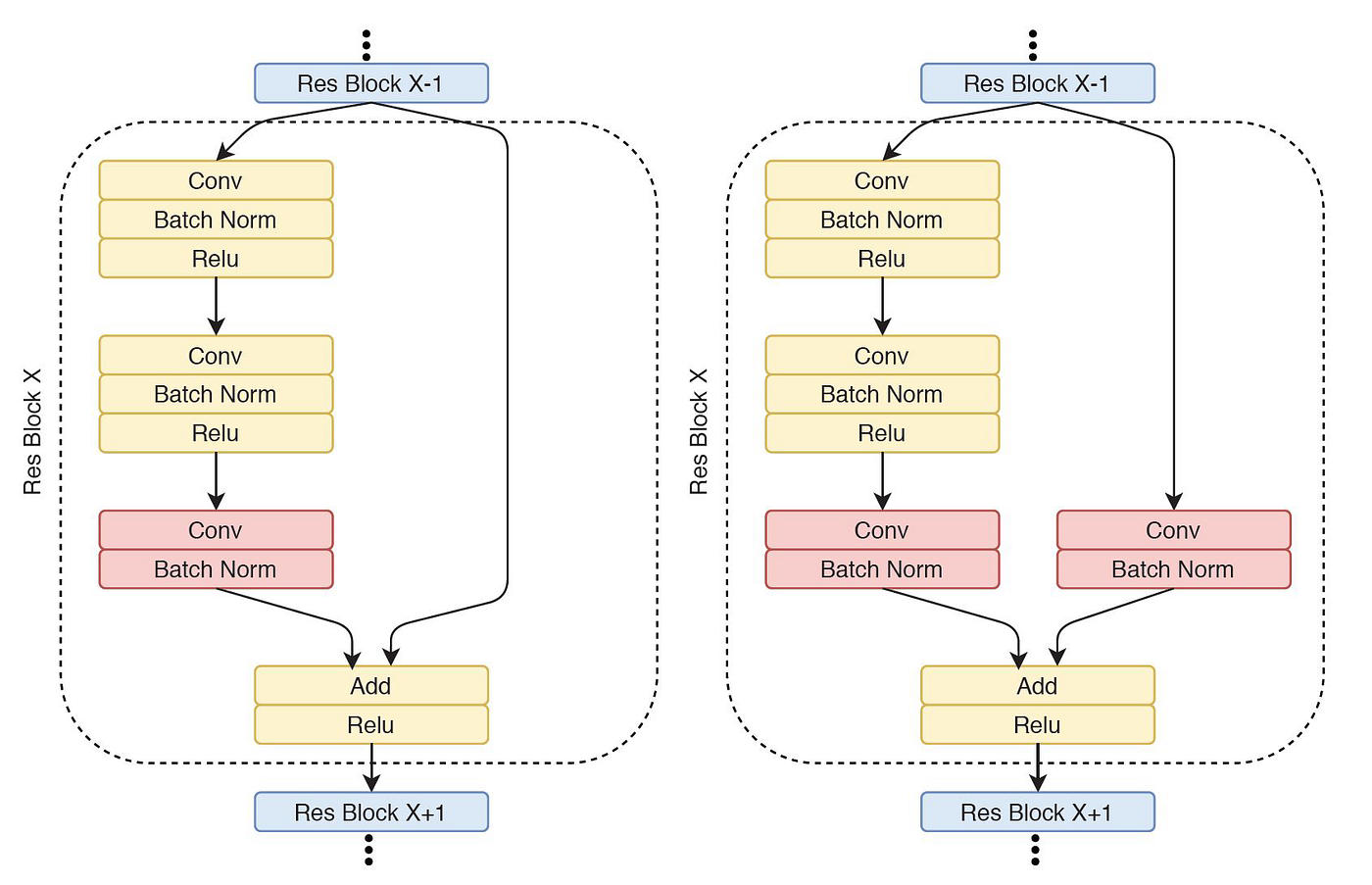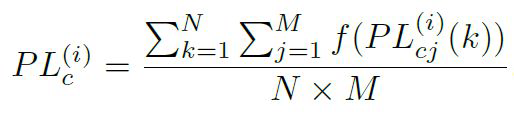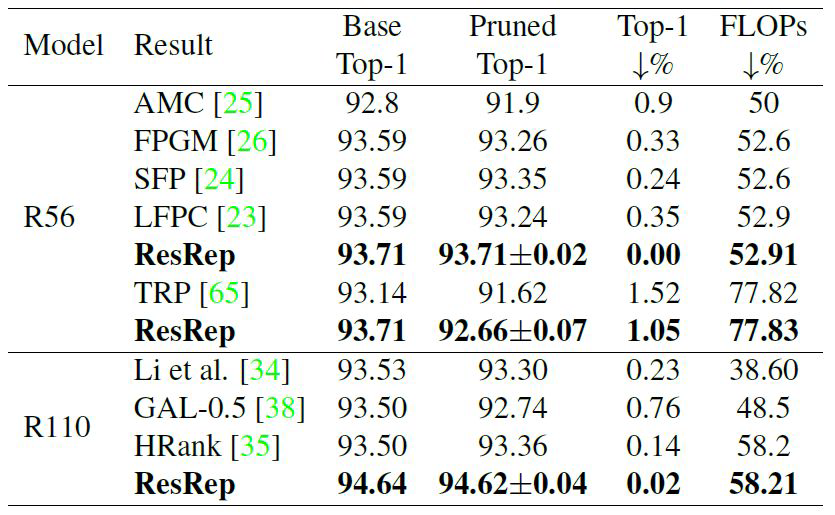# 1. Introduction

Deep neural networks, especially convolutional neural networks, are widely used in different tasks recently. However, different from traditional methods, deep learning requires a lot of storage space to store parameters in the network. Moreover, the inference time can be very long. These problems are more significant when people try to implement deep neural networks on edge devices which have small storage spaces and slow processing speeds. To deal with these problems, model compression is a very natural thought.

There are three methods for model compression:

○ Quantization

○ Weight Pruning

○ Knowledge Distillation

Parameter quantization is a very simple way to compress the model. It changes the parameters from floating-point numbers to low-bit width numbers. In other words, the parameters are changed from b bits to b’ bits, where b’ < b.

Weight pruning is a method to remove any parameters from the trained deep network model in order to get a more lightweight network while maintaining its accuracy. Both quantization and pruning try to find and remove the redundancy in the model parameters.

The purpose of knowledge distillation is to learn a distilled model (student network) and train this more compact neural network to reproduce the output from a larger network (teacher network). To train the student network, it can use not only the output predictions from the teacher network, but also the intermediate results.

In this blog, the focus is on how to compress CNN (Convolutional Neural Networks) by pruning and knowledge distillation. I will discuss two papers below: One is about the combination of pruning and knowledge distillation, and the other is a SOTA pruning method.

# 2. Combining Weight Pruning and Knowledge Distillation For CNN Compression

This paper proposed an available pruning method for ResNet and introduced a knowledge distillation architecture to do further compression. It is a very interesting way to combine weight pruning and knowledge distillation.

First is the pruning step. Before this paper, weight-pruning methods were mostly applied to basic and simple CNN architectures such as AlexNet and VGGNet since the deeper models, such as ResNet, have complex architectures which limit the application of pruning methods on them. However, this paper found a valid way to prune ResNet.

Considering the design concept of ResNet, pruning may destroy the dimensionality dependency between each pair of convolutional layers. In the bottom picture, we can see two different architectures for ResBlock, which is the most important part of ResNet. The layers in red have dimensionality dependency so they are un-prunable, and all other layers in yellow are prunable.Two different residual blocks in our ResNet50 model

This paper used the Average Percentage of Zeros (APoZ) to measure the importance of each neuron in a layer. The equation is shown below, where i is the layer index, c is the channel index, M is the total number of validation samples, and N is the dimension of the output feature map. Function f returns 1 if the variable within the bracket is equivalent to 0. If the APoZ of the neuron is larger than the standard deviation of the average APoZs in the same layer, then the neuron is prunable. We will keep pruning and fine-tuning until the accuracy drops significantly.The importance of the convolutional filter c in layer i

The second step is knowledge distillation (KD). Previously, there was a challenge to the KD methods: the number of layers and neurons in the student network is selected arbitrarily. As a result, the student network may be further compressed. Therefore, in this paper, the authors used the same model as the teacher network, but with fewer neurons in the un-prunable layers to make sure that the student network is entirely compressed.

Also, since the complex features are hard to learn, they implemented cosine similarities for not only the output layer, but also all intermediate layers as the loss function.

The results were shown through experiments for both regression and classification problems. For head-pose estimation, which is a regression task, they used ResNet50. For the classification task on the CIFAR10 dataset, they used ResNet110 and ResNet164. We can see that the combination of pruning and knowledge distillation compresses the model size significantly with only a slight decline in performance.

# 3. ResRep: Lossless CNN Pruning via Decoupling Remembering and Forgetting

This second paper solely talks about the pruning method. It proposed to re-parameterize a CNN into the remembering and forgetting parts, where the former learns to maintain the performance and the latter learns to prune.

The author proposed a term called perfect pruning, which means we only prune the parameters of pruned channels that are small enough, and the pruned model may still deliver the same performance as before. In order to do perfect pruning, we need two properties, resistance and prunability. We say a model has high resistance if the performance maintains high during training, and high prunability if the model endures a high pruning ratio with a low-performance drop.

An example of imperfect pruning would be the traditional penalty-based paradigm, which naturally suffers from a resistance-prunability trade-off. With the regularization part in the loss function, you cannot maintain the performance while pruning a lot of layers.

To solve this problem, this paper introduced Rep, which stands for Convolutional Reparameterization. For each convolutional layer, they append a compactor. Its kernel size is 1x1 and the number of kernels equals the number of channels. Also, its weights are initialized as an identity matrix. Therefore, its output is exactly as same as its input. After training all compactors, we can merge them with the convolutional layers easily with some transpose operations.

The code below is written using PyTorch. It proves that it is correct to append and merge compactors into convolutional layers. It should be noted that the output channels convM are equal to the output channels of convP, so pruning the output channel of the compactor is equivalent to pruning the output channel of the combined convolution layer.

In order to separately train the convolutional layers, the remembering part, and compactors, the forgetting part, this paper proposed Res, which stands for Gradient Resetting. Since we want convolutional layers only to focus on remembering, we use the original loss function without any regularization parts. But for the compactor, its gradient adds a part of regularization because we want it to learn how to forget. Since the objective function conflicts with the penalty, this paper used a binary mask to set the objective function to 0 for some channels for fast forgetting. For every 5 iterations, the model chooses several layers with the smallest weights to set their mask value to 0. And finally, after training processing, we can prune these layers and merge the pruned compactors and convolutional layers. This method is equivalent to directly pruning the convolutional layers.

The image below is the result of testing this pruning method on the CIFAR10 dataset. For both RestNet56 and ResNet110, ResRep achieved the smallest model with the highest accuracy.Pruning results of ResNet-56/110 on CIFAR-10

# 4. Insights and observations

For the first paper, I believe that the pruning method is not original. However, the idea of combining pruning and knowledge distillation is really interesting. Though the idea is pretty simple, it works well. Recently there have been more and more papers talking about using attention and discriminators to do knowledge distillation. However, this paper shows us that making the teacher model less redundant is more important than creating multiple complex loss functions. Also, this paper illustrates that multiple model compression methods can work better when implemented together.

Additionally, the experiment on the image classification problem used scratch student models. The result shows that the scratch models can perform well even without any further fine-tuning. Therefore, we can conclude that model designing is essential for deep learning problems.

For ResRep, I think the main idea is similar to dividing and conquering. It split the remembering and forgetting parts to attain perfect pruning. Since there are multiple trade-offs in deep learning problems, this idea could help us to decouple the problem so one does not affect the other.

Also, the author of this paper has published several papers about reparameterization, like RepVGG, which achieves higher accuracy than many transformers only using the normal VGG-styled model architecture. I believe this reparameterization method can be used in more fields in the future.

--

--